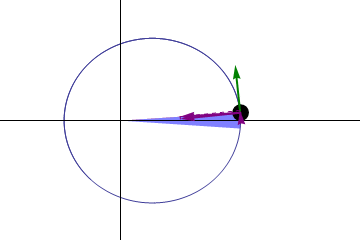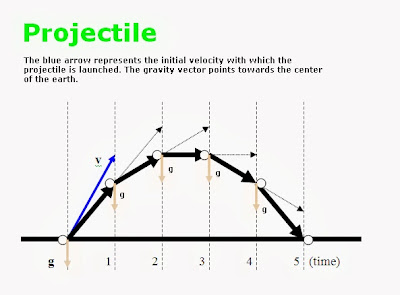### Angular Momentum - Part 2 [Kepler's Second Law]

By Johny Jagannath

[This blogpost has been updated into a chapter in this free eBook: The Story of Our Universe.]

So far, we have seen, with the help of a spinning top example, that the entity Angular Momentum is a fictitious entity, that points in a fictitious direction, and claims to be doing things that the centrifugal forces are doing.

We've also seen how the skater-girl is able to speed up her spinning rate by creating a new vector towards her by simply moving her hands towards her. This, therefore, takes us to the next logical step: Deriving the Kepler's Second Law, using the same techniques that we used before.

For those that don't know who Kepler is, he is the man most directly responsible for current theories of Gravity. [It amazes me that he was able to come up with such magnificent work despite the many adversities in his life].

At this point, I'm sure the reader is wondering why we are discussing The Kepler's Laws in the context of Angular Momentum. Let me explain why...

Since I have declared at the beginning of this article that Angular Momentum is a fictitious entity and explained the skater-girl's faster-spinning-rate in a new manner I am now going to have to explain, with the same reasoning, why the planets move faster when they are near the sun and slower when they are farther away. This seemingly unusual planetary motion is essentially the Kepler's Second Law.

This unusual motion of the planets has been generalized to all particles in the universe (including the skater girl), as a convention which physicists refer to as, The Conservation of Angular Momentum.

Perhaps it is unfair to not attach his name to this convention, but that is only my opinion and I don't know who coined the term.Kepler's Second Law.
Regardless, I will proceed to derive Kepler's Second Law, which is analogous to deriving the convention known as The Conservation of Angular Momentum, which we have seen so far to be a fictitious etity.

At, location 1, in the diagram to the left, we have a green sphere that represents the Earth and its initial velocity (or tangential velocity) given by the arrow, v

Our Sun's gravity pull is given by the arrow, g. Therefore using the parallelogram law, at location 1, we get the resultant whose direction and magnitude is given by, r.  Therefore the earth moves towards, the location 2.

At 2, the Earth's new velocity is given by r and the sun's gravity is given by, g. Using the parallelogram law again, we get a newer resultant whose magnitude and direction is indicated by the bold black arrow, which points towards the location 3. Therefore the earth moves toward the location 3, and so on.

Note that each parallelogram represents a unit of time [for example, each parallelogram is one second].

The triangles a, b and c, [and every one of the triangles formed out of the application of the parallelogram] will have equal areas. This is essentially Kepler's Second Law.

One can visually understand my diagram, by looking at the animation below (from Wikipedia). Please look at the green arrow, that becomes longer as it approaches the sun and shorter as it leaves away from the sun. My diagram's bold black arrows are essentially doing the same.Illustration of Kepler's second law. The planet moves faster near the Sun so the same area is swept out in a given time as at larger distances, where the planet moves more slowly. The green arrow represents the planet's velocity, and the purple arrows represents the force on the planet. [From Wikipeda]
Now that we've explained Kepler's Second Law, we have established the beginnings of a new theory of gravity, where gravity is not an accelerating force, but a bunch of particles that move at a constant velocity, towards the center of the Earth (or Sun). Anything that gets in the way, will get a slight push, that we experience as gravity, [denoted by the vector, g, in my diagram].Trajectory of a projectile
The big question is, why do gravity particles move toward the center of the Earth (or Sun)? I will answer that question later.

First, let us look at Projectiles and how the trajectory or path of a projectile can be traced using the same techniques that we used to derive Kepler's Second Law. Please view the diagram to the right, that illustrates how to trace the path of a projectile using the parallelogram law. For readers that might be interested in knowing whether my theory (of gravity) predicts the precession of elliptical orbits, then the answer is, yes.

Each orbit you trace using the parallelogram law (the way I have used it), will result in its orbit shifting and would resemble the animation shown below. Note that this is significantly different from Newton's Theory of Gravity.Planets revolving the Sun follow elliptical (oval) orbits that rotate gradually over time (apsidal precession). The eccentricity of this ellipse and the precession rate of the orbit are exaggerated for visualization. Most orbits in the Solar System have a much smaller eccentricity and precess at a much slower rate, making them nearly circular and stationary. [From Wikipedia]
With the explanation of gravity, I've covered explaining all important phenomena related to Angular Momentum and its conservation, except for the formation of our Solar System (and the science of Atoms), which I will discuss in a different post. For now, I will try to answer a question I had left unanswered, earlier. Recall, that I asked the big question: Why do gravity particles move towards the center of the Earth (or Sun)?

And the answer to that is, I have no idea. I can only make a guess that it probably has something to do with the Earth's core, where it is still hot and molten and full of activity. If and when I have a rational or a reasonable explanation I will post a blog-article, about it.